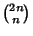## Sárközy's Theorem

A partial solution to the Erdös Squarefree Conjecture which states that the Binomial Coefficientis never Squarefree for all sufficiently large. Sárközy (1985) showed that ifis the square part of the Binomial Coefficient, thenwhereis the Riemann Zeta Function. An upper bound onofhas been obtained.

References

Erdös, P. and Graham, R. L. Old and New Problems and Results in Combinatorial Number Theory. Geneva, Switzerland: L'Enseignement Mathématique Université de Genève, Vol. 28, 1980.

Sander, J. W. A Story of Binomial Coefficients and Primes.'' Amer. Math. Monthly 102, 802-807, 1995.

Sárközy, A. On the Divisors of Binomial Coefficients, I.'' J. Number Th. 20, 70-80, 1985.

Vardi, I. Applications to Binomial Coefficients.'' Computational Recreations in Mathematica. Reading, MA: Addison-Wesley, pp. 25-28, 1991.